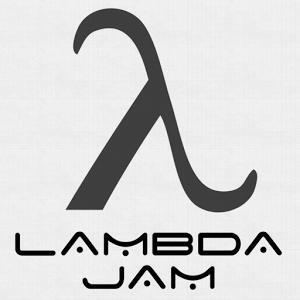# 编程经典案例之函数《Composing Software》一书有一章简单回顾了函数式编程的历史。

﻿

﻿

﻿

﻿

﻿

﻿

﻿

﻿

﻿

﻿

﻿

﻿

﻿

const addToCart = (cart, item, quantity) => {  cart.items.push({    item,    quantity  });  return cart;};const originalCart = {  items: []};const newCart = addToCart(  originalCart,  {    name: "Digital SLR Camera",    price: '1495'  },  1);

﻿

﻿

﻿

const addToCart = (cart, item, quantity) => {  return {    ...cart,    items: cart.items.concat([{      item,      quantity    }]),  };};

﻿

﻿

﻿

﻿

﻿

﻿

﻿

﻿

const doubleMap = numbers => {  const doubled = [];  for (let i = 0; i < numbers.length; i++) {    doubled.push(numbers[i] * 2);  }  return doubled;};

﻿

﻿

﻿

const doubleMap = numbers => numbers.map(n => n * 2);

﻿

﻿

﻿

2 者的区别，也可以类比语音聊天和文字聊天的区别，一个感性，凭直觉，效率高；一个理性，要思考，效率低。

﻿

﻿

﻿

﻿

const censor = words => {  const filtered = [];  for(let i = 0, { length } = words; i < length; i++) {    const word = words[i];    if (word.length !== 4) filtered.push(word)  }  return filtered;}const startsWithS = words => {  const filtered = [];  for(let i = 0, { length } = words; i < length; i++) {    const word = words[i];    if (word.startsWith('s')) filtered.push(word);  }  return filtered;}

﻿

﻿

﻿

﻿

const censor = words => filter(  word => word.length !== 4,  words);const startsWithS = words => filter(  word => word.startsWith('s'),  words);

﻿

﻿

const reduce = (reducer, initial, arr) => {  let acc = initial;  for(let i = 0, { length } = arr; i < length; i++) {    acc = reducer(acc, arr[i]);  }  return acc;}const filter=(  fn, arr) => reduce((acc,curr)=>fn(curr)?  acc.concat([curr]) :  acc, [], arr);

﻿

﻿

﻿

const highpass = cutoff => n => n >= cutoff;const gte3 = highpass(3);[1, 2 , 3, 4].filter(gte3); //[3,4];

﻿

﻿

﻿

﻿

﻿

﻿

﻿

function foo (/*parameters are declared here*/) {  //...}const foo = (/*parameters are declared here*/) => //...const foo = function(/*parameters are declared here*/) {  //...}

﻿

﻿

const add = a => b => a + b;

﻿

﻿

const result = add(2)(3); //=>5

﻿

﻿

const inc = add(1);inc(3);//=>4const inc10 = add(10);const inc20 = add(20);inc10(3);//=>13inc20(3);//=>23

﻿

﻿

﻿

﻿

﻿

const g = n => n + 1;const f = n => n * 2;const h = x => f(g(x));

﻿

﻿

const compose1 = (f,g) => x => f(g(x));const compose2 = (f,g) => x => g(f(x));...

﻿

﻿

﻿

const compose = (...fns) => x => fns.reduceRight((y, f)=>f(y), x);

﻿

﻿

const h = compose(f, g);

﻿

﻿

const trace = label => value => {  console.log(${ label }:${ value });  return value;}

﻿

﻿

const h = compose(  trace('after f'),  f,  trace('after g'),  g);

﻿

﻿## 评论